• 了解常见配方错误的原因。
• 排查高级公式字段。

语法错误

缺少或额外的括号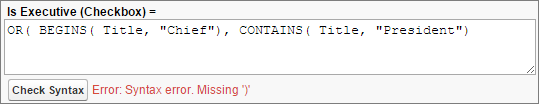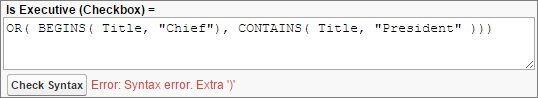字段不存在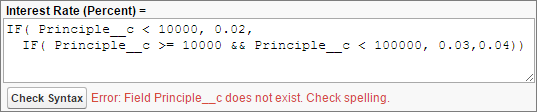参数数量不正确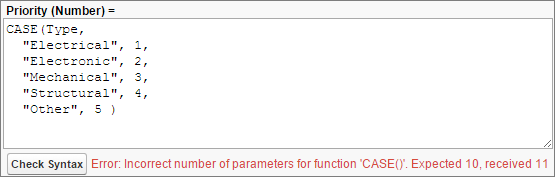未知功能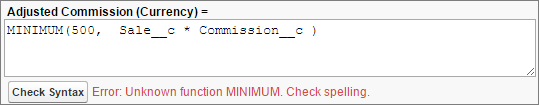使用不同的数据类型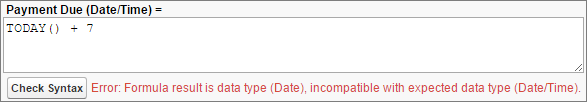编译大小和公式长度错误

编译大小限制

• Date1__c 是日期字段。
• Date2__c 是从Date1__c创建日期的公式字段。
DATE( YEAR( Date1__c ), MONTH( Date1__c ), DAY( Date1__c ) )
下面的公式返回给定日期的月份的最后一天的日期（假设2月总是有28天）：
DATE(
YEAR( SomeDate__c ),
MONTH( SomeDate__c ),
IF(
OR(
MONTH( SomeDate__c ) = 4,
MONTH( SomeDate__c ) = 6,
MONTH( SomeDate__c ) = 9,
MONTH( SomeDate__c ) = 11
),
30,
IF(
MONTH( SomeDate__c ) = 2,
28,
31
)
)
)

DATE(
YEAR( SomeDate__c ),
MONTH( SomeDate__c ),
CASE(
MONTH( SomeDate__c ),
2, 28,
4, 30,
6, 30,
9, 30,
11, 30,
31
)
)

CASE( Agent__c,
"John", Base_Commission__c * 2,
"Jane", Base_Commission__c * 6,
/* 重复许多其他代理 */
Base_Commission__c
)

Base_Commission__c * CASE( Agent__c,
"John", 2,
"Jane", 6,
/* 许多其他代理重复 */
1
)

学习目标

• 实现高级公式以匹配复杂的用例。
• 写干净，易于理解的公式。

把它写出来

1. 在安装程序中，使用快速查找框来查找 Object Manager.
2. 点击 Opportunity | Validation Rules.
3. 点击 New.
4. Rule Name,输入 Loss Reason Required.
5. Error Condition Formula, 输入以下公式：
AND(
ISCHANGED(StageName),
ISPICKVAL(StageName, "Closed Lost"),
ISPICKVAL(Loss_Reason__c, "")
)
6. Error Message, 输入 Opportunities can’t be marked Closed Lost without providing a Loss Reason.
7. 对于错误位置，请选择 Field 和 Loss Reason.
8. 点击 Save.

使用清除间距和格式

IF(AND(AnnualRevenue > 1000000, CONTAINS(CASE(Country,
"United States", "US", "America", "US", "USA", "US", "US", "US",

IF(
AND(AnnualRevenue > 1000000,
CONTAINS(CASE(Country, "United States", "US", "America",
"US", "USA", "US", "US", "US", "NA"), "US")),
IF(
IF(
OR(
), "Warm”, "Cold"
)
), "Cold"
)

IF(
AnnualRevenue > 1000000 &&
CONTAINS(CASE(Country, "United States", "US", "America", "US",
"USA", "US", "US", "US", "NA"), "US"),
IF(
IF(
"Warm", "Cold"
)
), "Cold"
)

把事情简单化

OR (
CloseDate < DATE( YEAR(TODAY()), MONTH(TODAY()), 1),
IF (
AND (
MONTH (TODAY() ) =1,
CloseDate > DATE( YEAR(TODAY() ), MONTH(TODAY() ), 31)),
true,
IF (
AND (
MONTH (TODAY() ) =2,
CloseDate > DATE( YEAR(TODAY() ), MONTH(TODAY() ), 28)),
true,
IF (
AND (
MONTH (TODAY() ) =3,
CloseDate > DATE( YEAR(TODAY() ), MONTH(TODAY() ), 31)),
true,
IF (
AND (
MONTH (TODAY() ) =4,
CloseDate > DATE( YEAR(TODAY() ), MONTH(TODAY() ), 30)),
true,
IF (
AND (
MONTH (TODAY() ) =5,
CloseDate > DATE( YEAR(TODAY() ), MONTH(TODAY() ), 31)),
true,
IF (
AND (
MONTH (TODAY() ) =6,
CloseDate > DATE( YEAR(TODAY() ), MONTH(TODAY() ), 30)),
true,
IF (
AND (
MONTH (TODAY() ) =7,
CloseDate > DATE( YEAR(TODAY() ), MONTH(TODAY() ), 31)),
true,
IF (
AND (
MONTH (TODAY() ) =8,
CloseDate > DATE( YEAR(TODAY() ), MONTH(TODAY() ), 31)),
true,
IF (
AND (
MONTH (TODAY() ) =9,
CloseDate > DATE( YEAR(TODAY() ), MONTH(TODAY() ), 30)),
true,
IF (
AND (
MONTH (TODAY() ) =10,
CloseDate > DATE( YEAR(TODAY() ), MONTH(TODAY() ), 31)),
true,
IF (
AND (
MONTH (TODAY() ) =11,
CloseDate > DATE( YEAR(TODAY() ), MONTH(TODAY() ), 30)),
true,
IF (
AND (
MONTH (TODAY() ) =12,
CloseDate > DATE( YEAR(TODAY() ), MONTH(TODAY() ), 31)),
true, false
)))))))))))))

NOT(
AND(
MONTH( CloseDate ) = MONTH( TODAY() ),
YEAR( CloseDate ) = YEAR( TODAY() )
)
)

学习目标

• 描述什么是文本类型。
• 将文本值转换为其他数据类型或从其他数据类
• 描述使用文本类型的公式的用例。
• 使用文本类型创建一个公式。

公式中的文本介绍

FirstName & " " & LastName

常见的文本函数和操作符

Salesforce带有许多功能和操作员，可以使用公式中的文本进行工作。使用公式，可以将其他数据类型转换为文本并将文本值连接在一起，或在文本字段中搜索特定的字符串。

转换为文本值和从文本值转换

TEXT（）将百分比，数字，日期，日期/时间，选取列表或货币字段转换为文本。 TEXT（）返回没有任何格式，逗号或货币符号的输出。例如，TEXT（percent_value），如果percent_value设置为30％，则返回0.3。

其他文字功能

Salesforce包含其他用于处理公式中文本和格式化的函数。有关更多信息，请参阅Salesforce联机帮助中的公式运算符和函数。

在公式中使用文本类型

分配客户评级

• Cold—客户不符合任何这些条件。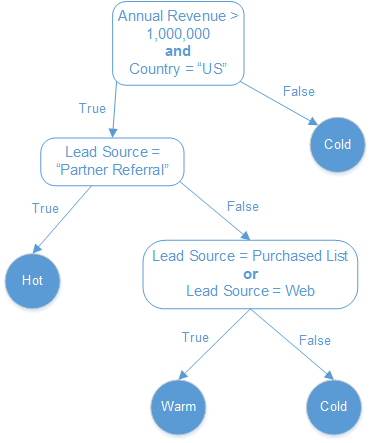1. 在安装程序中，使用快速查找框来查找 Object Manager.
2. 点击 Lead | Fields & Relationships 然后单 New.
3. 选择Formula 并单击 Next.
4. Field Label, 输入 Rating. 字段名称自动填充。
5. 选择 Text 并单击 Next.
6. 输入以下公式：
IF(
AnnualRevenue > 1000000 &&
CONTAINS(CASE(Country, "United States", "US", "America", "US",
"USA", "US", "US", "US", "NA"), "US"),
IF(
IF(
"Warm", "Cold"
)
), "Cold"
)

用CASE（）显示图像

IMAGE（）函数最多需要四个参数。 IMAGE（image_url，alternate_text，height，width）以可选的height和width参数指定的尺寸显示由image_url指定的图像。如果图像无法显示，则该功能显示alternate_text。

• Hot, 公式显示五颗星
• Warm,公式显示三颗星
• Cold, 公式显示一颗星

IMAGE(
CASE(Rating__C,
"Hot", "/img/samples/stars_500.gif",
"Warm", "/img/samples/stars_300.gif",
"Cold", "/img/samples/stars_100.gif",
"/img/samples/stars_000.gif"),
"Unknown")

文本示例

1. 此公式使用美国客户的帐单州/省字段将其分类为北，南，东，西或中央。
IF(ISBLANK(BillingState), "None",
IF(CONTAINS("AK:AZ:CA:HA:NV:NM:OR:UT:WA", BillingState), "West",
IF(CONTAINS("CO:ID:MT:KS:OK:TX:WY", BillingState), "Central",
IF(CONTAINS("CT:ME:MA:NH:NY:PA:RI:VT", BillingState), "East",
IF(CONTAINS("AL:AR:DC:DE:FL:GA:KY:LA:MD:MS:NC:NJ:SC:TN:VA:WV", BillingState), "South",
IF(CONTAINS("IL:IN:IA:MI:MN:MO:NE:ND:OH:SD:WI", BillingState), "North", "Other"))))))
3. 此公式接受Email字段并使用它来创建一个URL。该公式使用FIND（）和SUBSTITUTE（）在电子邮件地址中查找@符号，并用www替换它的左侧所有内容。
SUBSTITUTE(Email, LEFT(Email, FIND("@", Email)), "www.")
文本公式的常见错误

公式字段帮手

IF(((NOT(IsClosed)) && (TODAY() - DATEVALUE(CreatedDate) > 20)), "RED",
IF((NOT(IsClosed)) && (TODAY() - DATEVALUE(CreatedDate) > 10), "YELLOW",
"GREEN"))

TODAY() - DATEVALUE(CreatedDate)

IF(((NOT(IsClosed)) && (Case_Age_In_Days__c > 20)), "RED",
IF((NOT(IsClosed)) && (Case_Age_In_Days__c > 10), "YELLOW",
"GREEN"))